Mobile QR Code1. (Department of Computer Engineering, Dankook University / Yongin, Korea)
2. (Department of Software and Computer Engineering, Ajou University / Suwon, Korea )

Wireless passive sensor network, Interference power, Asymptotic normality, Normal approximation, Berry-Esséen bound, Test for normality

## 1. Introduction

A wireless passive sensor network is a kind of wireless sensor network, which consists of sink nodes, sensor nodes, and notably radio frequency (RF) sources . In a wireless passive sensor network, an RF source radiates RF waves and supplies energy to sensor nodes. In turn, a sensor node receives the RF waves and obtains energy. Consuming the energy, the sensor node senses the environment, gathers information and produces a packet. Then, the sensor node transmits the packet to a sink node. Theoretically, an RF source is able to continuously supply abundant energy to sensor nodes. In practice, however, an RF source is only permitted to transmit a limited amount of power. Furthermore, the RF waves radiated by an RF source experiences a heavy path loss en route to a sensor node. Also, the received RF waves are not efficiently converted to direct currents at the sensor node. Against theoretical expectations, a sensor node suffers from a scarcity of energy in a wireless passive sensor network [2,3].

A typical wireless passive sensor network consists of a single sink node and a coexisting single RF source. Due to a scarcity of energy, the wireless passive sensor network provides only limited coverage, where a relatively small number of sensor nodes are geographically scattered around the sink node. In such a configuration, two or more sensor nodes can simultaneously attempt to deliver their packets to the sink node [2,3]. Thus, a medium access control (MAC) scheme is needed for the sensor nodes to succeed in packet delivery. For some reasons, e.g., scarcity of energy and difficulty in signaling, the wireless passive sensor network opts to employ a contending-type MAC scheme, for example, framed and slotted ALOHA .

In a wireless passive sensor network governed by a contending-type MAC scheme, two or more sensor nodes may send their packets to a sink node simultaneously. Then, the packets collide  and the sink node may not be able to identify any of them. In a wireless passive sensor network, sensor nodes are not usually equidistant from a sink node. Thus, when some packets collide with each other, the sink node receives them with mutually different power levels. If the power level of a packet is sufficiently higher than the interference power, i.e., the power level of interfering packets, the sink node may be able to identify the packet. Such a phenomenon, called capture , makes a significant impact on the performance of a wireless passive sensor network.

As explained above, it is necessary to characterize the interference power to evaluate the performance of a wireless passive sensor network. Then, the characteristics of the channel from a sensor node to a sink node should be specified a priori since a packet experiences path loss while traveling from a sensor node to a sink node. There have been a number of reports on the channel characteristics in wireless sensor networks [6-10]. They considered a variety of environments; agricultural greenhouses, underground pipes, and rice fields. However, most of them commonly assumed a log-distance path loss model  and focused on calibrating the parameters involved in the log-distance path loss model. The log-distance path loss model contains a term that expresses the effect of shadowing, which is represented by a random variable governed by a log-normal distribution. Then, the interference power in a wireless sensor network can also be represented by a sum of log-normal random variables.

The exact distribution function of a sum of log-normal random variables is not in a tractable form so that it is not easy to calculate the signal-to-interference ratio (SIR), for example. Naturally, many efforts have been made to approximate a sum of log-normal random variables. Such efforts are largely classified into two categories. One is the category of approximations that focus on enhancing the accuracy [12-21] and the other is the category of approximations which are more concerned with tractability [22-24]. In , Fenton considered a log-normal random variable, which has the same mean and variance as a sum of log-normal random variables, and proposed it to approximate the sum. In , Marlow showed that a sum of log-normal random variables asymptotically has a normal distribution by using the Lindeberg-Feller central limit theorem . Then, he suggested a normal approximation to a sum of log-normal random variables. In , Bkassiny considered multiple-input multiple-output free space optical systems and introduced an Erlang approximation to a sum of log-normal random variables. As far as we know, however, there has been no work on approximating the interference power, which can be modeled as a sum of log-normal random variables, in the unique environment of wireless passive sensor networks.

In this paper, we consider a wireless passive sensor network in which a number of sensor nodes deliver their packets to a sink node according to a contending-type MAC scheme. In the network, two or more sensor nodes may simultaneously send their packets to a sink node, which incurs a collision among the packets. Then, the delivery of a packet may be hampered by the other packets involved in the collision since the power of the packet can be overwhelmed by the interference power, i.e., the power brought by the interfering packets. The interference power highly influences the performance of the wireless passive sensor network. Thus, it is of necessity to appropriately model the interference power to evaluate the performance of the wireless passive sensor network. First, we assume that a packet suffers a log-distance path loss  while traveling from a sensor node to a sink node. Then, we characterize the interference power as a sum of log-normal random variables. Secondly, we prove the asymptotic normality of the interference power by using the Lyapunov central limit theorem . Based on the property of asymptotic normality, pursuing tractability rather than accuracy, we propose a normal approximation to the interference power brought by a finite number of sensor nodes. Thirdly, as a measure for the accuracy of normal approximation, we take the Kolmogorov-Smirnov distance  between the exact and approximate distribution functions of the interference power. Then, we develop an exact expression of the Berry-Esséen bound [27-29], which is in fact an upper bound on the Kolmogorov-Smirnov distance, associated with the interference power. Fourthly, we employ three kinds of statistical tests for normality; the Kolmogorov-Smirnov test , the Cramér-von Mises test [31,32] and the Anderson-Darling test . Then, we evaluate the acceptability of the normal approximation to the interference power.

The main contributions of this paper are as follows.

▪ First, we describe the power brought by interfering packets in a wireless passive sensor network employing a contending-type MAC scheme.

▪ Secondly, by using the Lyapunov central limit theorem, we prove the asymptotic normality of the interference power, i.e., the property that the interference power converges in distribution to a normal random variable as the number of interfering packets goes to infinity.

▪ Thirdly, we consider Kolmogorov-Smirnov distance between the distribution function of the interference power and the proposed normal distribution function. Then, we derive the exact Berry-Esséen bound associated with the interference power in a closed form. In addition, we develop an exact expression of the Berry-Esséen bound associated with the sum of normal, uniform, shifted exponential, or Pareto random variables which are mutually independent but are not necessarily distributed identically.

▪ Fourthly, we adopt three well-known statistical tests for normality; the Kolmogorov-Smirnov test, the Cramér-von Mises test and the Anderson-Darling test. Then, we investigate the acceptability of the proposed normal approximation to the interference power occurring in a wireless passive sensor network.

The rest of the paper is organized as follows. In Section 2, we characterize the interference power in a wireless sensor network ruled by a contending-type MAC scheme. In Section 3, we prove the asymptotic normality of the interference power and we also propose a normal approximation to the interference power. In Section 4, we derive the Berry-Esséen bound associated with the interference power and carry out a comparative evaluation of the accuracy of the normal approximation. Section 5 is devoted to statistical testing for the normality of the interference power, which provides evidence that the normal approximation to the interference power is acceptable. In Section 6, we consider log-normal and Erlang approximations besides the normal approximation. Then, we compare the approximate distribution functions with the empirical distribution function of the interference power. In Section 7, we suppose that a specific contending-type MAC scheme is employed by a wireless passive sensor network. Then, we investigate the accuracy of the throughput value calculated by using the normal approximation.

## 2. Interference Power

Consider a typical wireless passive sensor network which consists of a single sink node, a single RF source and $M$ sensor nodes, denoted by $s_{1},\cdots ,s_{M}$. As illustrated in Fig. 1, the RF source is positioned near the sink node while the sensor nodes are scattered around the sink node. Let $D_{m}$ denote the distance between the sink node and sensor node $s_{m}$ for $m\in \left\{1,\cdots ,M\right\}$. In practice, distance $D_{m}$ is bounded from above as well as from below, i.e., there exist $D_{U}$ and $D_{L}$ such that

##### (1)
0 < D L D m D U <

for all $m\in \left\{1,\cdots ,M\right\}$.

Theoretically, a sink node is able to supply abundant energy to sensor nodes in a wireless passive sensor network. Against theoretical expectations, however, a sensor node suffers from a scarcity of energy in practice. As a result, a sensor node is not able to directly power itself with the received energy but has to temporarily store it at a capacitor, for example. Inevitably, a sensor node needs a time interval for charging its capacitor prior to transmitting a packet. For wireless passive sensor networks, a MAC scheme is thus devised in consideration of such a scarcity of energy [2,3]. In the wireless passive sensor network, we suppose that time is divided into frames and each frame is partitioned again into a charging interval and a transmitting interval. (See Fig. 2) During a charging interval, the RF source radiates RF waves. Then, a sensor node receives the RF waves, converts them to direct current (DC) and charges its internal capacitor. As a transmitting interval begins, a sensor node senses the environment, gathers information and encapsulates the data into a packet. During the transmitting interval, the sensor node then transmits the packet to the sink node according to a contending-type MAC scheme, e.g., framed and slotted ALOHA .

Assume that all the sensor nodes are equipped with same energy harvester, capacitor charger and packet transmitter. Suppose that every sensor node transmits a packet with same power. Let $U$ denote the amount of power transmitted by a sensor node. Assume that each charging interval is long enough so that even the farthest sensor node from the sink node is able to charge its capacitor as sufficiently as it can construct a packet and then transmit the packet with power $U$. Even if two sensor nodes transmit their packets with same power, the sink node does not necessarily receive these packets with same power since the packets experience different path losses . There have been several research works on the channel characteristics in wireless sensor networks [6-10]. They were conducted in a wide variety of environments; agricultural greenhouses, rice fields and suburbs. However, many of them focused on calibrating the parameters involved in the log-distance path loss model . Also, a wireless passive sensor network has some unique characteristics related to the fading that happens in the signal from a sensor node to the sink node; a sensor node transmits a relatively narrowband signal to the sink node, a sensor node has almost no mobility and the distance between the sink node and a sensor node is relatively short. Thus, frequency-selective fading and Doppler shift rarely take place in a wireless passive sensor network. Furthermore, the multipath fading is not as significant as the shadow fading in a wireless passive sensor network.

Let $V_{m}$ denote the power with which the sink node receives a packet from sensor node $s_{m}$ for $m\in \left\{1,\cdots ,M\right\}$. Then, based on the argument above, we set that received power $V_{m}$ conforms to the log-distance path loss model. Then, received power $V_{m}$ is related to transmitted power $U$ as follows.

##### (2)
+ 10 γ log 10 D 0 10 γ log 10 D m + Z m

in decibels and

##### (3)
V m = U K D 0 D m γ e 1 10 log 10 Z m

in watts, where $K$ is the path loss constant, $D_{0}$ is the reference distance, $\gamma$ is the path loss exponent, and $Z_{m}$ is a random variable governed by a normal distribution with mean $0$ and variance $\omega ^{2}$. Also, assume that random variables $Z_{1},\cdots ,Z_{M}$ are mutually independent. Set

##### (4)
α = 1 10 log 10 β m = log U m K D 0 D m γ

for $m\in \left\{1,\cdots ,M\right\}$. Then, $V_{m}$ can be rewritten by

##### (5)
V m = e α Z m + β m

for $m\in \left\{1,\cdots ,M\right\}$. Note that $Z_{m}$ has the normal distribution with mean $0$ and variance $\omega ^{2}$ so that $\alpha Z_{m}+\beta _{m}$ also has the normal distribution with mean $\beta _{m}$ and variance $\alpha ^{2}\omega ^{2}$. Thus, $V_{m}$ is governed by the log-normal distribution whose density

##### (6)
f m x = 1 2 π α 2 ω 2 x e log x β m 2 2 α 2 ω 2

for $x\in \left(0,\infty \right)$. Also, the mean and variance of $V_{m}$ are as follows.

##### (7)
E V m = e 1 2 α 2 ω 2 + β m V a r V m = e α 2 ω 2 1 e α 2 ω 2 + 2 β m .

For m ˜ 1, , M , suppose that sensor node s m ˜ transmits a packet during a transmitting interval. Assume that other n 1, , M 1 sensor nodes simultaneously transmit their packets during the same transmitting interval. Let s π 1 , , s π n denote these n sensor nodes, where 1 π 1 < < π n M and π k 1, , M m ˜ for all k 1, , n . Then, the packets from s π 1 , , s π n collide with the packet from s m ˜ and thus interfere with the packet from s m ˜ . Let X n denote the power brought to the sink node by the interfering packets. Then, X n can be expressed by

##### (8)
X n = k = 1 n V π k .

In conclusion, the interference power is expressed by the sum of log-normal random variables which are mutually independent but are not necessarily identical.

## 3. Asymptotic Normality of and Normal Approximation to Interference Power

In Section 2, the interference power $X_{n}$ is expressed by a sum of log-normal random variables. Unfortunately, a sum of log-normal random variables has neither distribution function nor density in a tractable form. To easily calculate the ratio of signal to interference and noise (SINR), for example, we suggest a normal approximation to the interference power. Also, we show the validity of the normal approximation by proving that interference power $X_{n}$ converges to a normal random variable in distribution.

As shown in (8), interference power $X_{n}$ is the sum of mutually independent random variables, denoted by $V_{{\pi _{1}}},\cdots ,V_{{\pi _{M}}}$. However, they are not necessarily distributed identically. Nonetheless, the Lyapunov central limit theorem  says that interference power $X_{n}$ converges to a normal random variable as far as the Lyapunov condition  is satisfied. The following lemma shows that random variables $V_{{\pi _{1}}},V_{{\pi _{2}}},\cdots$ satisfy the Lyapunov condition. Also, the theorem below confirms that interference power $X_{n}$ consequently converges to a normal random variable in distribution according to the Lyapunov central limit theorem.

$\textbf{Lemma 1}$ There exists $\delta >0$ such that

##### (9)
$\frac{\sum _{k=1}^{n}E\left(\left| V_{{\pi _{k}}}-E\left(V_{{\pi _{k}}}\right)\right| ^{2+\delta }\right)}{\left[\sqrt{\sum _{k=1}^{n}Var\left(V_{{\pi _{k}}}\right)}\right]^{2+\delta }}\rightarrow 0$

as $n\rightarrow \infty$.

Proof: A proof is given in the appendix.

$\textbf{Theorem 1}$ Set

##### (10)
$\overset{˜}{X}_{n}=\frac{1}{\sqrt{\sum _{k=1}^{n}Var\left(V_{{\pi _{k}}}\right)}}\sum _{k=1}^{n}\left[V_{{\pi _{k}}}-E\left(V_{{\pi _{k}}}\right)\right].$

Let $\overset{˜}{F}_{n}$ denote the distribution function of $\overset{˜}{X}_{n}$. Set $W$ to be a random variable having the standardized normal distribution. Let $\Phi$ denote the distribution function of $W$, i.e.,

##### (11)
$\Phi \left(y\right)=\int _{-\infty }^{y}\frac{1}{\sqrt{2\pi }}e^{-\frac{1}{2}{x^{2}}}dx$

for $y\in \mathrm{\mathbb{R}}$. Then,

##### (12)
$\overset{˜}{X}_{n}\overset{d}{\rightarrow }W$

as $n\rightarrow \infty$, i.e.,

##### (13)
$\overset{˜}{F}_{n}\left(x\right)\rightarrow ~ \Phi \left(x\right)$

for every $x\in \mathrm{\mathbb{R}}$.

Proof: A proof is given in the appendix.

From Theorem 1, interference power $X_{n}$ can be approximated by a random variable $Y_{n}$, which is

##### (14)
$Y_{n}=\sqrt{\sum _{k=1}^{n}Var\left(V_{{\pi _{k}}}\right)}W+\sum _{k=1}^{n}E\left(V_{{\pi _{k}}}\right)$

for $n\in \mathrm{\mathbb{N}}$.

## 4. Berry-Esséen Bound Associated with Interference Power

This section is devoted to investigating the similarity between the interference power $X_{n}$ defined in (8) and the normal random variable $Y_{n}$ defined in (14). A way of investigating the accuracy of an approximate distribution function of the interference power is to examine the distance or metric between the exact and approximate distribution functions. Many kinds of distances have been proposed, e.g., the total variation distance , the Wasserstein metric , the Lévy metric , and the Kolmogorov-Smirnov distance . Among them, the Kolmogorov-Smirnov distance is known to have a considerable advantage of computational simplicity . Also, a useful bounding technique is available for the Kolmogorov-Smirnov distance . Thus, we first adopt the Kolmogorov-Smirnov distance to measure the accuracy of the normal approximation. Next, we derive an exact expression of the Berry-Esséen bound [27-29], which is an upper bound on the Kolmogorov-Smirnov distance, associated with the interference power. Finally, we carry out a comparative study of the Berry-Esséen bound associated with the interference power.

### 4.1 Derivation of Berry-Esséen Bound Associated with Interference Power

Recall the normalized interference power, denoted by $\overset{˜}{X}_{n}$ in (10). Note that it has mean $0$ and variance $1$. Remind that $W$ denotes a random variable having the standardized normal distribution. Then, the Kolmogorov-Smirnov distance between $\overset{˜}{X}_{n}$ and $W$ is defined by}

##### (15)
$d_{KS}\left(\overset{˜}{X}_{n},W\right)=\sup \left\{\left| \overset{˜}{F}_{n}\left(x\right)-\Phi \left(x\right)\right| \colon x\in \mathrm{\mathbb{R}}\right\}$

where $\overset{˜}{F}_{n}$ and $\Phi$ are distribution functions of $\overset{˜}{X}_{n}$ and $W$, respectively. Apparently, a small value of $d_{KS}\left(\overset{˜}{X}_{n},W\right)$ indicates strong similarity between $\overset{˜}{X}_{n}$ and $W$. Define

##### (16)
$b_{BE}\left(X_{n}\right)=C_{0}\frac{\sum _{k=1}^{n}E\left(|V_{{\pi _{k}}}-E\left(V_{{\pi _{k}}}\right)|^{3}\right)}{\left[\sum _{k=1}^{n}Var\left(V_{{\pi _{k}}}\right)\right]^{\frac{3}{2}}}$

where $C_{0}$ is a positive constant. Then, $b_{BE}\left(X_{n}\right)$, which is the Berry-Esséen bound associated with interference power $X_{n}$, is an upper bound on the Kolmogorov-Smirnov distance between $\overset{˜}{X}_{n}$ and $W$, i.e.,

##### (17)
$d_{KS}\left(\overset{˜}{X}_{n},W\right)\leq b_{BE}\left(X_{n}\right)$

for all $n\in \mathrm{\mathbb{N}}$. Thus, it can be said that a small value of $b_{BE}\left(X_{n}\right)$ tends to indicate a short Kolmogorov-Smirnov distance and hence strong similarity between $\overset{˜}{X}_{n}$ and $W$. The following theorem exactly calculates the Berry-Esséen bound associated with interference power $X_{n}$.

$\textbf{Theorem 2}$ The Berry-Esséen bound associated with $X_{n}$
##### (18)
\begin{aligned} b_{B E}\left(X_{n}\right)=& \frac{C_{0} \sum_{k=1}^{n} e^{3 \beta_{\pi_{k}}}}{e^{\alpha^{3} \omega^{3}}\left[e^{\alpha^{2} \omega^{2}}-1\right]^{\frac{3}{2}}\left[\sum_{k=1}^{n} e^{2 \beta_{\pi_{k}}}\right]^{\frac{3}{2}}} \\ & \times\left[e^{\frac{9 \alpha^{2} \omega^{2}}{2}}\left[2 \Phi\left(\frac{5 \alpha \omega}{2}\right)-1\right]\right.\\ &-3 e^{\frac{5 \alpha^{2} \omega^{2}}{2}}\left[2 \Phi\left(\frac{3 \alpha \omega}{2}\right)-1\right] \\ &+4 e^{\frac{3 \alpha^{2} \omega^{2}}{2}}\left[2 \Phi\left(\frac{\alpha \omega}{2}\right)-1\right] \end{aligned}

Proof: A proof is given in the appendix.

### 4.2 Evaluation of Berry-Esséen Bound Associated with Interference Power

For a comparative evaluation of the Berry-Esséen bound associated with the interference power, we introduce a random variable $S_{n}$, which is a sum of random variables as follows.}

##### (19)
$S_{n}=\sum _{k=1}^{n}T_{k}$

where random variables $T_{1},\cdots ,T_{n}$ are not necessarily distributed identically but are mutually independent. For all $k\in \left\{1,\cdots ,n\right\}$, we then consider the four cases below. In Case 1, $T_{k}$ has the normal distribution with mean $\mu _{k}$ and variance $\sigma _{k}^{2}$, i.e., the density of $T_{k}$

##### (20)
$g_{k}\left(x\right)=\frac{1}{\sqrt{2\pi \sigma _{k}^{2}}}e^{-\frac{1}{2\sigma _{k}^{2}}{\left(x-\mu _{k}\right)^{2}}}$

for $x\in \mathrm{\mathbb{R}}$. In Case 2, $T_{k}$ has the uniform distribution in $\left[a_{k},b_{k}\right]$, i.e., the density of $T_{k}$

##### (21)
$g_{k}\left(x\right)=\frac{1}{b_{k}-a_{k}}$

for $x\in \left(a_{k},b_{k}\right)$. In Case 3, $T_{k}$ has the exponential distribution with scale parameter $\delta _{k}$ and rate parameter $\lambda _{k}$, i.e., the density of $T_{k}$

##### (22)
$g_{k}\left(x\right)=\lambda _{k}e^{-{\lambda _{k}}\left(x-\delta _{k}\right)}$

for $x\in \left(\delta _{k},\infty \right)$. In Case 4, $T_{k}$ has the Pareto distribution with scale parameter $\delta _{k}$ and shape parameter $\nu _{k}$, i.e., the density of $T_{k}$

##### (23)
$g_{k}\left(x\right)=\frac{\nu _{k}\delta _{k}^{\nu _{k}}}{x^{{\nu _{k}}+1}}$

for $x\in \left(\delta _{k},\infty \right)$. The theorem below shows the Berry-Esséen bounds associated with $S_{n}$ in these four cases.

$\textbf{Theorem 3}$ In the four cases above, the Berry-Esséen bounds associated with $S_{n}$ are as follows.

If $T_{k}$ has the normal distribution with mean $\mu _{k}$ and variance $\sigma _{k}^{2}$,

##### (24)
$b_{BE}\left(S_{n}\right)=\frac{2\sqrt{2}C_{0}\sum _{k=1}^{n}\sigma _{k}^{3}}{\sqrt{\pi }\left[\sum _{k=1}^{n}\sigma _{k}^{2}\right]^{\frac{3}{2}}}.$

If $T_{k}$ has the uniform distribution in $\left[a_{k},b_{k}\right]$,

##### (25)
$b_{BE}\left(S_{n}\right)=\frac{3\sqrt{3}C_{0}\sum _{k=1}^{n}\left(b_{k}-a_{k}\right)^{3}}{4\left[\sum _{k=1}^{n}\left(b_{k}-a_{k}\right)^{2}\right]^{\frac{3}{2}}}.$

If $T_{k}$ has the exponential distribution with scale parameter $\delta _{k}$ and rate parameter $\lambda _{k}$,

##### (26)
$b_{BE}\left(S_{n}\right)=\frac{2\left(6e^{-1}-1\right)C_{0}\sum _{k=1}^{n}\frac{1}{\lambda _{k}^{3}}}{\left[\sum _{k=1}^{n}\frac{1}{\lambda _{k}^{2}}\right]^{\frac{3}{2}}}.$

If $T_{k}$ has the Pareto distribution with scale parameter $\delta _{k}$ and shape parameter $\nu _{k}$,

##### (27)
\begin{aligned} b_{B E}\left(S_{n}\right)=& \frac{C_{0}}{\left[\sum_{k=1}^{n} \frac{\delta_{k}^{2} v_{k}}{\left(v_{k}-1\right)\left(v_{k}-2\right)}\right]^{\frac{3}{2}}} \\ & \times \sum_{k=1}^{n}\left[\frac{12 \delta_{k}^{3}}{\left(v_{k}-1\right)\left(v_{k}-2\right)\left(v_{k}-3\right)}\left(1-\frac{1}{v_{k}}\right)^{v_{k}-3}\right.\\ &\left.-\frac{2 \delta_{k}^{3} v_{k}\left(v_{k}+1\right)}{\left(v_{k}-1\right)^{3}\left(v_{k}-2\right)\left(v_{k}-3\right)}\right] \end{aligned}

Proof: A proof is given in the appendix.

Using the formulae in theorems 2 and 3, we carry out a numerical comparison of $b_{BE}\left(X_{n}\right)$ with $b_{BE}\left(S_{n}\right)$. In the comparison, we suppose that the wireless passive sensor network is configured as a honeycomb. As illustrated in Fig. 3, the coverage of the network consists of 19 disks. The boundary of each disk then circumscribes one of the non-overlapping 19 hexagons. A sink node lies on the disk which is at the center of the coverage. On the other hand, a sensor node resides in each of the 18 disks except the central disk. Besides the network configuration, various physical parameters are involved in the numerical comparison. Their values are summarized in Table 1.

With respect to the number of interfering packets, Fig. 4 shows the average Berry-Esséen bound, which is yielded under some assumptions below. Since the wireless passive sensor network consists of 18 sensor nodes and each sensor node transmits at most one packet during a transmitting interval, the number of interfering packets can be a number in $\left\{1,\cdots ,17\right\}$. Suppose that the number of interfering packets is $n\in \left\{1,\cdots ,17\right\}$. Then, the sensor nodes that sent such interfering packets form an $\textit{n}$-combination of $\left\{s_{1},\cdots ,s_{18}\right\}$. Note that the number of $\textit{n}$-combinations is equal to $\left(\begin{array}{l}18\\n\end{array}\right)$. In Fig. 4, we first assume that the set of the sensor nodes whose packets create interference is an $\textit{n}$-combination of $\left\{s_{1},\cdots ,s_{18}\right\}$ equally likely, i.e., with probability $\frac{1}{\left(\begin{array}{l}18\\n\end{array}\right)}$. Then, we exhibit the expected Berry-Esséen bound with respect to the set of interfering sensor nodes. In this figure, $S_{n}$ is a sum of normal, uniform, exponential, or Pareto random variables, denoted by $T_{1},\cdots ,T_{n}$ as in (19). Secondly, we then assume that $T_{k}$ has the same mean and variance as $V_{{\pi _{k}}}$ has for every $k\in \left\{1,\cdots ,n\right\}$. The exact value of positive constant $C_{0}$ appearing in the Berry-Esséen bound has not been found up to now. However, a number of upper bounds on the constant have been reported. Among them, the smallest upper bound is known to be 0.56 . Thirdly, we assume that constant $C_{0}$ is 0.56 in Fig. 4.

Table 1. Values of parameters.

 Parameter Value Transmitted power $\textit{U}$ 1 W Path loss constant $\textit{K}$ 0.000570 Reference distance $\textit{D}_0$ 1 m Distance to sensor node $\textit{D}_k$ $\sqrt{3}$,2, 2$\sqrt{3}$ m Path loss exponent γ 2 Standard deviation ω 1 dB

In Fig. 4, we observe that the interference power incurs a higher Berry-Esséen bound than a sum of normal or uniform random variables. Note that a sum of normal random variables is also normally distributed. On the other hand, the interference power produces a lower Berry-Esséen bound than a sum of exponential or Pareto random variables. Such an observation reveals the tendency that the interference power is more similar to a normal random variable than a sum of exponential or Pareto random variables, wheares it is less similar than a sum of normal or uniform random variables. In addition, we notice that the average Berry-Esséen bound monotonically decreases as the number of interfering packets increases. This notice confirms that the interference power is apt to converge to a normal random variable as more packets create interference.

Fig. 3. Network configuration in numerical comparison of Berry-Esséen bounds.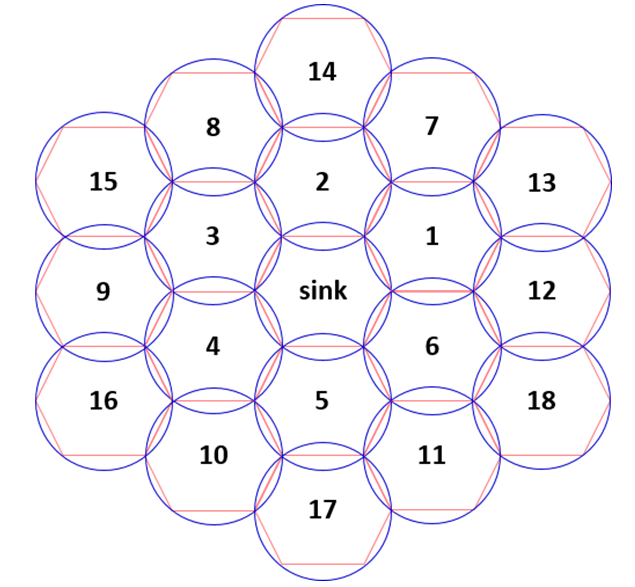Fig. 4. Average Berry-Esséen bound vs. number of interfering packets.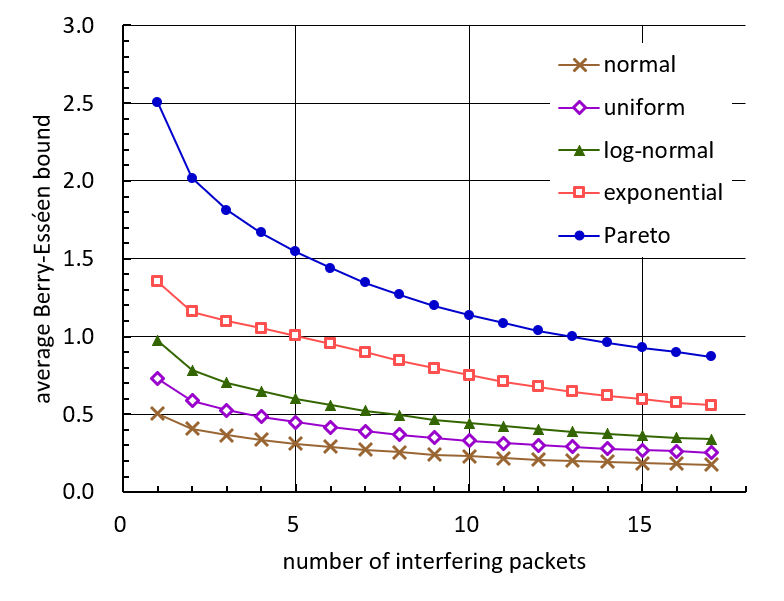## 5. Test for Normality of Interference Power

In this section, we statistically test the normality of the interference power. Specifically, we employ three kinds of well-known tests; the Kolmogorov-Smirnov test , the Cramér-von Mises test [31,32] and the Anderson-Darling test .}

Recall that $\overset{˜}{X}_{n}$ and $W$ represent the normalized interference power and a standardized normal random variable, respectively. Also, note that $\overset{˜}{F}_{n}$ and $\Phi$ denote the distribution functions of $\overset{˜}{X}_{n}$ and $W$, respectively. To test the normality of the interference power, we construct null and alternative hypotheses, denoted by $\mathrm{H}_{0}$ and $\mathrm{H}_{1}$, as follows.

##### (28)
$\mathrm{H}_{0}\colon \overset{˜}{F}_{n}=\phi$ $\mathrm{H}_{1}\colon \overset{˜}{F}_{n}\neq \phi$

Then, we test the null hypothesis $\mathrm{H}_{0}$. For such a test, we adopt the Kolmogorov-Smirnov test, the Cramér-von Mises test and the Anderson-Darling test, which are commonly based on an empirical distribution function . Suppose that we obtain $J$ samples of normalized interference power $\overset{˜}{X}_{n}$. Let $\overset{˜}{X}_{n,1},\cdots ,\overset{˜}{X}_{n,J}$ denote the samples. Also, let $\overset{˜}{X}_{n,\left(1\right)},\cdots ,\overset{˜}{X}_{n,\left(J\right)}$ denote the order statistics of $\overset{˜}{X}_{n,1},\cdots ,\overset{˜}{X}_{n,J}$ such that $\overset{˜}{X}_{n,\left(1\right)}\leq \cdots \leq \overset{˜}{X}_{n,\left(J\right)}$ almost surely. Then, an empirical distribution function of $\overset{˜}{X}_{n}$ is defined by

##### (29)
$\overset{˜}{H}_{n}\left(x\right)=\frac{1}{J}\sum _{j=1}^{J}I_{\left\{\overset{˜}{X}_{n,\left(j\right)}\leq x\right\}}$

for $x\in \mathrm{\mathbb{R}}$. Note that empirical distribution function $\overset{˜}{H}_{n}$ is an estimator of true distribution function $\overset{˜}{F}_{n}$.

First, the Kolmogorov-Smirnov test uses the supremum distance between $\overset{˜}{H}_{n}$ and $\Phi$. Set

##### (30)
$U_{\left(j\right)}=\phi \left(\overset{˜}{X}_{n,\left(j\right)}\right)$

for $j\in \left\{1,\cdots ,J\right\}$. Then, its test statistic $KS_{J}$ is expressed by

##### (31)
$KS_{J}=\max \left\{KS_{J}^{+},KS_{J}^{-}\right\}$

where

##### (32)
\begin{aligned} K S_{J}^{+} &=\max \left\{\frac{j}{J}-U_{(j)}: j \in\{1, \cdots, J\}\right\} \\ K S_{J}^{-} &=\max \left\{U_{(j)}-\frac{j-1}{J}: j \in\{1, \cdots, J\}\right\} . \end{aligned}

A smaller value of test statistic $KS_{J}$ indicates a higher similarity between $\overset{˜}{F}_{n}$ and $\Phi$. If the value of $KS_{J}$ is higher than a prescribed critical value, the Kolmogorov-Smirnov test rejects null hypothesis $\mathrm{H}_{0}$.

Secondly, the Cramér-von Mises test uses a quadratic distance between $\overset{˜}{H}_{n}$ and $\Phi$. Its test statistic $CM_{J}$ is expressed by

##### (33)
$CM_{J}=\sum _{j=1}^{J}\left[U_{\left(j\right)}-\frac{2j-1}{2J}\right]^{2}+\frac{1}{12J}.$

A smaller value of test statistic $CM_{J}$ indicates a higher similarity between $\overset{˜}{F}_{n}$ and $\Phi$. If the value of $CM_{J}$ is higher than a prescribed critical value, the Cramér-von Mises test rejects null hypothesis $\mathrm{H}_{0}$.

Thirdly, the Anderson-Darling test uses a weighted quadratic distance between $\overset{˜}{H}_{n}$ and $\Phi$. Its test statistic $AD_{J}$ is expressed by

##### (34)
\begin{aligned} A D_{J}=&-J-\frac{1}{J} \sum_{j=1}^{J}(2 j-1) \log \left(U_{(j)}\right) \\ &-\frac{1}{J} \sum_{j=1}^{J}(2 J-2 j+1) \log \left(1-U_{(j)}\right) \end{aligned}

A smaller value of test statistic $AD_{J}$ indicates a higher similarity between $\overset{˜}{F}_{n}$ and $\Phi$. If the value of $AD_{J}$ is higher than a prescribed critical value, the Anderson-Darling test rejects the null hypothesis $\mathrm{H}_{0}$.

Table 2. Interfering sensor nodes.

 Number of interfering packets Interfering sensor nodes 2 $s_{1}$, $s_{2}$ 5 $s_{1}$, $s_{2}$, $s_{7}$, $s_{8}$, $s_{13}$ 8 $s_{1}$, $s_{2}$, $s_{3}$, $s_{7}$, $s_{8}$, $s_{9}$, $s_{13}$, $s_{14}$ 11 $s_{1}$, $s_{2}$, $s_{3}$, $s_{4}$, $s_{7}$, $s_{8}$, $s_{9}$, $s_{10}$, $s_{13}$, $s_{14}$, $s_{15}$ 14 $s_{1}$, $s_{2}$, $s_{3}$, $s_{4}$, $s_{5}$, $s_{7}$, $s_{8}$, $s_{9}$, $s_{10}$, $s_{11}$, $s_{13}$, $s_{14}$, $s_{15}$, $s_{16}$ 17 $s_{1}$, $s_{2}$, $s_{3}$, $s_{4}$, $s_{5}$, $s_{6}$, $s_{7}$, $s_{8}$, $s_{9}$, $s_{10}$, $s_{11}$, $s_{12}$, $s_{13}$, $s_{14}$, $s_{15}$, $s_{16}$, $s_{17}$

Figs. 5 and 6 demonstrate empirical distribution functions constructed from the samples of the normalized interference power. Meanwhile, Figs. 7-9 show the results of Kolmogorov-Smirnov, Cramér-von Mises and Anderson-Darling tests for the normality of interference power. In these figures, we suppose a wireless passive sensor network, which consists of a single sink node and 18 sensor nodes, as illustrated in Fig. 3. We also assume that the parameters involved in the path loss model take the values appearing in Table 1. In addition, we suppose that the interference is created by 2, 5, 8, 11, 14, or 17 packets, which are sent by the sensor nodes listed in Table 2. For each number of interfering packets, we generate 1000 samples of the normalized interference power and then perform the three tests for normality.

In each of Figs. 5 and 6, empirical distribution functions of the normalized interference power, denoted by $\overset{˜}{F}_{n}$ are compared with the distribution function of standardized normal random variable, denoted by $\Phi$. We suppose that the interference is created by 2 packets in Fig. 5, whereas it is induced by 17 packets in Fig. 6. In these figures, we observe that both empirical distribution functions are similar to the distribution function of the standardized normal random variable. In addition, we notice that $\overset{˜}{F}_{n}$ is more similar to $\Phi$ when 17 packets create interference than when 2 packets induce interference.

Fig. 7 shows the values of the Kolmogorov-Smirnov test statistic with respect to the number of interfering packets. In this figure, these values of the test statistic are compared with the critical values at the 0.01, 0.05, 0.1, and 0.2 levels of significance. In this figure, we observe that the value of the Kolmogorov-Smirnov test statistic is lower than the critical value at the 0.2 level of significance when the number of interfering packets is 8, 11, 14, or 17. Thus, null hypothesis $\mathrm{H}_{0}$, which proposes that $\overset{˜}{F}_{n}=\phi$, cannot be rejected at the significance level of 0.2. In other words, we cannot say that the interference power does not have a normal distribution. In this figure, we also notice that the value of the test statistic is lower than the critical value at the 0.05 level of significance when 2 packets induce interference. Thus, from such a notice, $\mathrm{H}_{0}$ cannot be rejected at the 0.05 level of significance.

Fig. 5. Empirical distribution function vs. normal distribution function (Number of interfering packets = 2).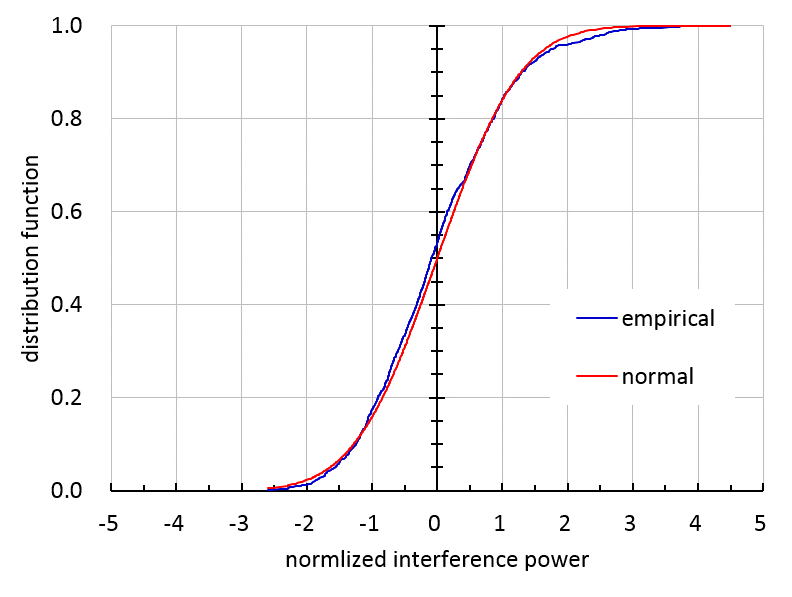Fig. 6. Empirical distribution function vs. normal distribution function (Number of interfering packets = 17).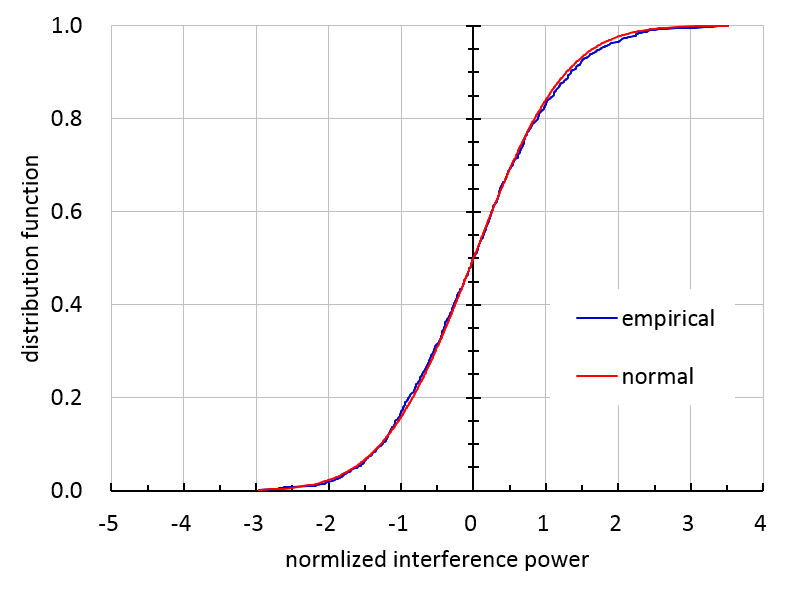Fig. 7. Kolmogorov-Smirnov test for normality of interference power.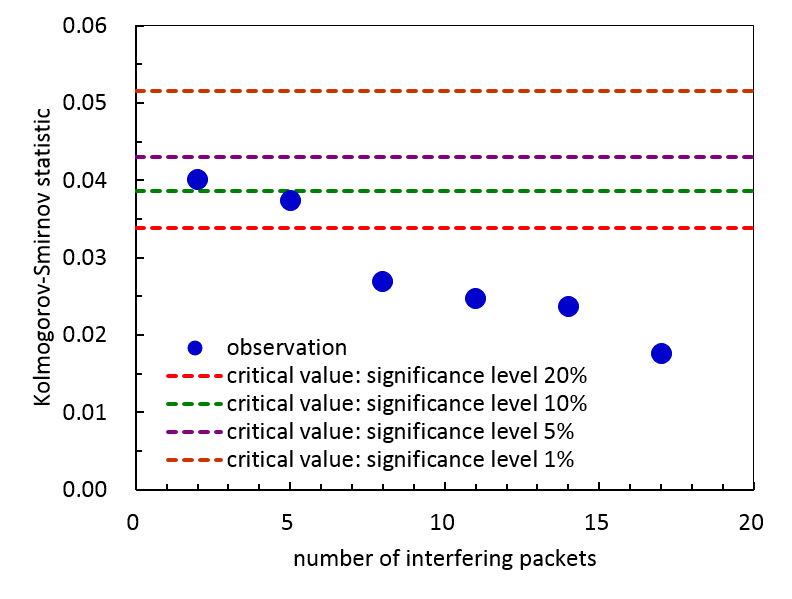Fig. 8. Cramér-von Mises test for normality of interference power.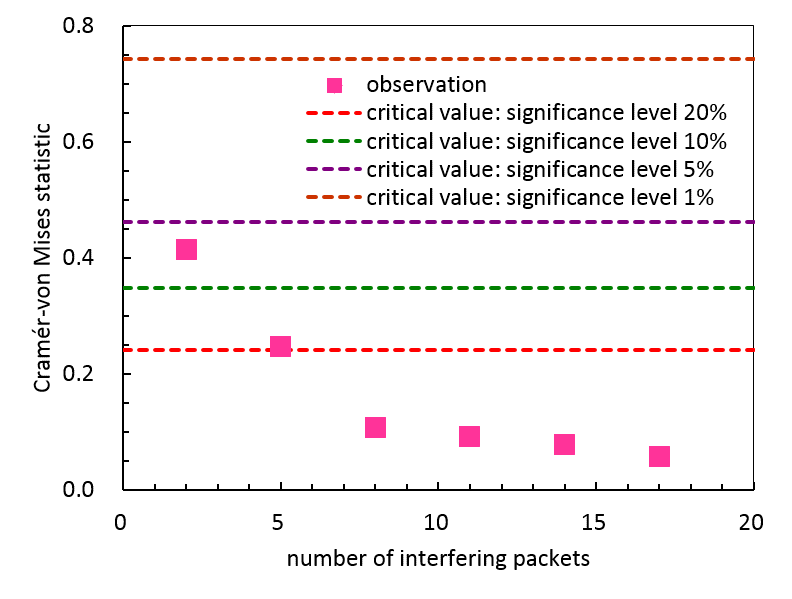Fig. 9. Anderson-Darling test for normality of interference power.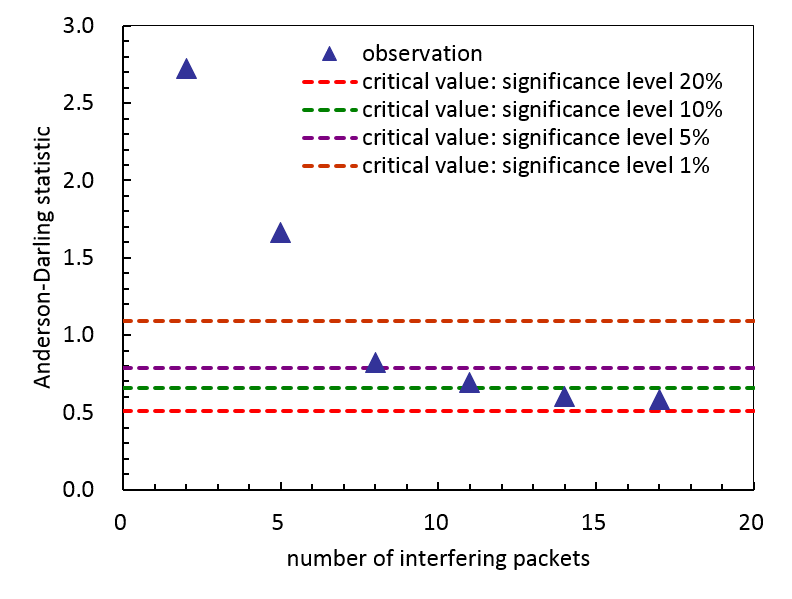Fig. 8 shows the values of the Cramér-von Mises test statistic with respect to the number of interfering packets. The tendency expressed by the test results in Fig. 8 is similar to the one exhibited in Fig. 7. When the number of interfering packets is 8, 11, 14, or 17, the value of the test statistic is lower than the critical value at the 0.2 level of significance. Thus, the null hypothesis $\mathrm{H}_{0}$ cannot be rejected at the significance level of 0.2. When the number of interfering packets is 2, the value of the test statistic is also lower than the critical value at the 0.05 level of significance so that $\mathrm{H}_{0}$ cannot be rejected.

Fig. 9 shows the values of the Anderson-Darling test statistic with respect to the number of interfering packets. In this figure, we observe that the value of the test statistic is lower than the critical value at the 0.05 level of significance when the number of interfering packets is 8, 11, 14, or 17. Thus, null hypothesis $\mathrm{H}_{0}$ cannot be rejected at the significance level of 0.05. However, when the number of interfering packets is 2, the value of the test statistic is higher than the critical value at the 0.01 level of significance. Thus, $\mathrm{H}_{0}$ can be rejected at the significance level of 0.01.

In conclusion, most of the test results confirm that the null hypothesis $\mathrm{H}_{0}$ cannot be rejected at the 0.05 level of significance, i.e., we cannot say that the interference power does not have a normal distribution. Also, the test results explicitly reveal the tendency that $\mathrm{H}_{0}$ cannot be rejected at higher level of significance as the interference is created by more packets.

## 6. Comparison of Approximate Distribution Functions of Interference Power

In this section, we consider log-normal and Erlang approximations in  and  in addition to the normal approximation. Then, we compare the approximate distribution functions with the empirical distribution function of the interference power.

Figs. 10 and 11 compare 3 approximate distribution functions (normal, log-normal and Erlang distribution functions) with the empirical distribution function of the interference power when the number of interfering packets is 2 and 17, respectively. In these figures, we assume that the wireless passive sensor network is configured as illustrated in Fig. 3 and is characterized by the parameter values as listed in Table 1. Also, the interfering sensor nodes are selected according to Table 2. Figs. 10 and 11 demonstrate that each of the normal, log-normal and Erlang approximations yield a distribution function similar to the empirical distribution function of the interference power. Also, each approximation exhibits no meaningful difference between empirical and approximate distribution functions.

Fig. 10. Comparison of approximate distribution functions with empirical distribution function of interference power when packets of 2 sensor nodes interfere.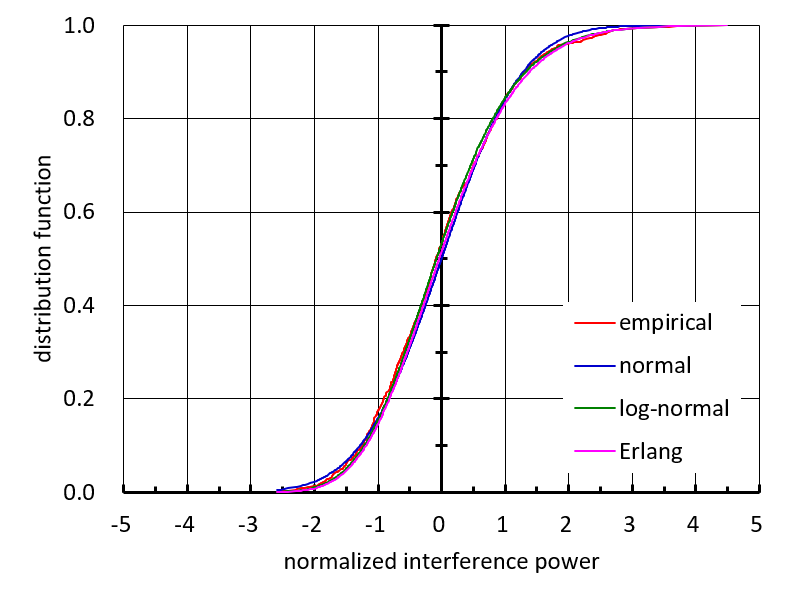Fig. 11. Comparison of approximate distribution functions with empirical distribution function of interference power when packets of 17 sensor nodes interfere.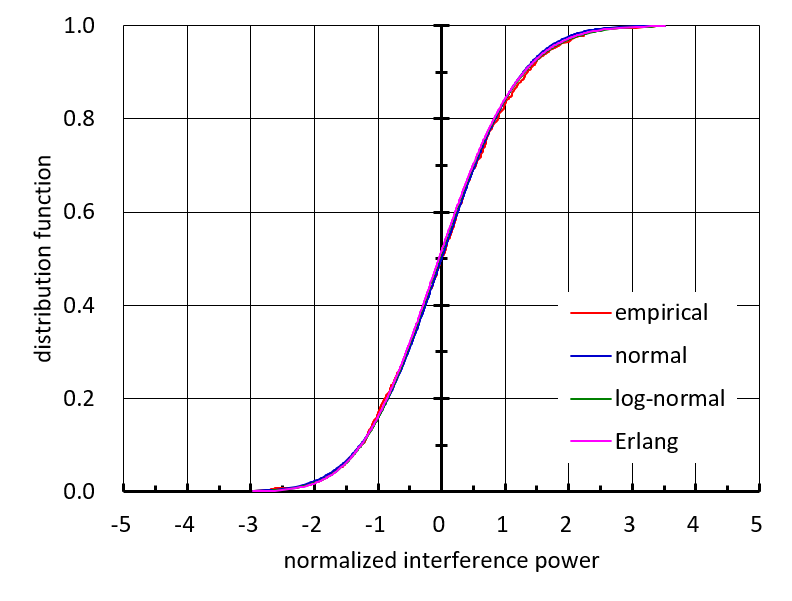Fig. 12. Comparison of exact and approximate values of throughput.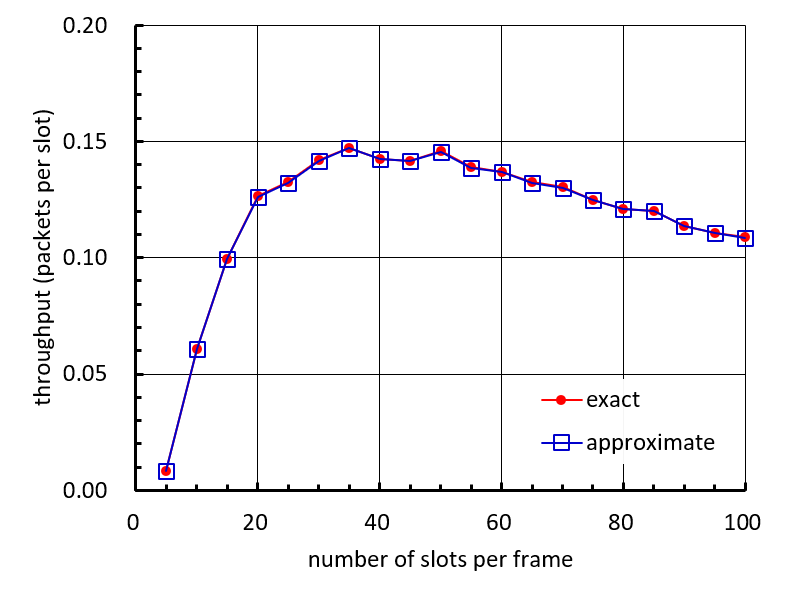## 7. Investigation of Throughput Value Yielded by Normal Approximation

In this section, we focus on the throughput that the wireless passive sensor network is able to achieve and examine the accuracy of the throughput value yielded by the normal approximation.

Fig. 12 shows the exact and approximate values of the throughput with respect to the number of slots per frame. In this figure, we suppose that the wireless passive sensor network is configured as illustrated in Fig. 3 and is governed by the parameter values listed in Table 1. Also, we assume that the wireless passive sensor network employs the contending-type MAC scheme addressed in . The MAC scheme uses the same time structure as depicted in Fig. 2, where each transmitting interval consists of a number of slots and each charging interval perdures for 40 slot times. In every frame, each sensor node equally likely selects a slot among the slots that comprise the transmitting interval of the frame. Then, the sensor node transmits a packet during the selected slot. In Fig. 12, both of the exact and approximate throughput curves are obtained by use of a simulation method, where the approximate throughput curve is yielded by the normal approximation to the interference power. Fig. 12 reveals that the approximate value of the throughput barely differs from the exact value regardless of the number of slots per frame.

## 8. Conclusion

We considered a wireless passive sensor network in which sensor nodes deliver their packets to a sink node according to a contending-type MAC scheme. In the network, a packet collision inevitably takes place and the interference power brought by the colliding packets may hinder a sink node from identifying any packet. Apparently, the interference power has a strong influence on the performance of a wireless passive sensor network so that it is of necessity to appropriately describe the interference power for performance evaluation. In this paper, we characterized the interference power as a sum of random variables governed by log-normal distributions, which are mutually independent but are not necessarily identical. Then, we proved the asymptotic normality of the interference power and, in pursuit of tractability rather than accuracy, proposed a normal approximation to the interference power. First, as a measure for the accuracy of the proposed normal approximation, we chose the Kolmogorov-Smirnov distance and presented an exact expression of the Berry-Esséen bound associated with the interference power. Comparative studies of Berry-Esséen bounds implied that the normal approximation to the interference power is moderately accurate. Next, to explore the acceptability of the proposed normal approximation, we employed three well-known statistical tests for normality. Hypothesis testing revealed that the normal approximation is acceptable even in the case that the interference power is incurred by a relatively small number of sensor nodes in a wireless passive sensor network.

### REFERENCES

1
Akan O., Isik M., Baykal B., August 2009, Wireless Passive Sensor Networks, IEEE Communications Magazine, Vol. 47, No. 8, pp. 92-992
Seo H., Park J., Choi C., August 2017, Simple Contending-type MAC Scheme for Wireless Passive Sensor Networks: Throughput Analysis and Optimization, IEIE Transactions on Smart Processing and Computing, Vol. 6, No. 4, pp. 222-3043
Seo H., Park J., Choi C., April 2020, Timeliness-aware Anti-discrimination MAC Scheme for Wireless Passive Sensor Networks, IEIE Transactions on Smart Processing and Computing, Vol. 9, No. 2, pp. 151-1684
Schoute F., April 1983, Dynamic frame length ALOHA, IEEE Transactions on Communications, Vol. 31, No. 4, pp. 565-5685
Rom R., Sidi M., 1990, Multiple Access Protocols - Performance and Analysis., New York, USA: Springer-Verlag6
Martínez-Sala A., Molina-Garcia-Pardo J., Egea-López E., Vales-Alonso J., Juan Llacer L., García-Haro J., December 2005, An Accurate Radio Channel Model for Wireless Sensor Network Simulation, Journal of Communications and Networks, Vol. 7, No. 4, pp. 401-4077
Greenberg E., Sheinberg A., April 2015, Statistical Channel Model for Wireless Sensor Networks Deployment in Suburban Environment, Proceedings of 9th European Conference on Antennas and Propagation (EuCAP 2015), Lisbon, Portugal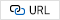8
Yang G., Gan X., Chen H., December 2016, Radio Channel Model for Agricultural Wireless Sensor Networks, Proceedings of 3rd International Conference on Wireless Communication and Sensor Network (WCSN 2016), Wuhan, China, pp. 708-7129
Mekid S., Wu D., Hussain R., Youcef-Toumi K., November 2017, Channel Modeling and Testing of Wireless Transmission for Underground In-pipe Leak and Material Loss Detection, International Journal of Distributed Sensor Networks, Vol. 13, No. 1110
Gao Z., Li W., Zhu Y., Tian Y., Pang F., Cao W., Ni J., 2018, Wireless Channel Propagation Characteristics and Modeling Research in Rice Field Sensor Networks, Sensors, Vol. 18, No. 911
Agrawal D., Zeng Q., 2015, Introduction to Wireless and Mobile Systems., 4th edition, Boston, USA: Cengage Learning12
Janos W., May 1970, Tail of the Distribution of Sums of Log-normal Variates, IEEE Transactions on Information Theory, Vol. 16, No. 3, pp. 299-30213
Schleher D., March 1977, Generalized Gram-Charlier Series with Application to the Sum of Log-normal Variates, IEEE Transactions on Information Theory, Vol. 23, No. 2, pp. 275-28014
Schwartz S., Yeh Y., September 1982, On the Distribution Function and Moments of Power Sums with log-normal Components, Bell System Technical Journal, Vol. 61, No. 7, pp. 1441-146215
Beaulieu N., Xie Q., March 2004, An Optimal Lognormal Approximation to Lognormal Sum Distributions, IEEE Transactions on Vehicular Technology, Vol. 53, No. 2, pp. 479-48916
Lam C., Le-Ngoc T., July 2007, Log-shifted Gamma Approximation to Lognormal Sum Distributions, IEEE Transactions on Vehicular Technology, Vol. 56, No. 4, pp. 2121-212917
Liu Z., Almhana J., Wang F., McGorman R., September 2007, Mixture Lognormal Approximations to Lognormal Sum Distributions, IEEE Communications Letters, Vol. 11, No. 9, pp. 711-71318
Nie H., Chen S., October 2007, Lognormal Sum Approximation with Type IV Pearson Distribution, IEEE Communications Letters, Vol. 11, No. 10, pp. 790-79219
Liu Z., Almhana J., McGorman R., July 2008, Approximating Lognormal Sum Distributions with Power Lognormal Distributions, IEEE Transactions on Vehicular Technology, Vol. 57, No. 4, pp. 2611-261720
Li X., Wu Z., Chakravarthy V., Wu Z., October 2011, A Low-complexity Approximation to Lognormal Sum Distributions via Transformed Log Skew Normal Distribution, IEEE Transactions on Vehicular Technology, Vol. 60, No. 8, pp. 4040-404521
Cobb B., Rumí R., Salmerón A., September 2012, Approximating the Distribution of a Sum of Log-normal Random Variables, Proceedings of 6th European Workshop on Probabilistic Graphical Models (PGM 2012), Granada, Spain, pp. 67-7422
Fenton L., March 1960, The Sum of Log-normal Probability Distributions in Scatter Transmission Systems, IRE Transactions on Communications Systems, pp. 57-6723
Marlow N., November 1967, A Normal Limit Theorem for Power Sums of Independent Random Variables, Bell System Technical Journal, Vol. 46, No. 9, pp. 2081-208924
Bkassiny M., May 2009, Approximation of Log-normal Sum and Rayleigh Sum Distributions Using the Erlang Distribution, Proceedings of 8th Faculty of Engineering and Architecture Student Conference (FEASC 2009), Beirut, Lebanon25
Karr A., 1993, Probability., New York, USA: Springer-Verlag26
Lehmann E., Romano J., 2005, Testing Statistical Hypotheses. 3rd edition, New York, USA: Springer27
Berry A., 1941, The Accuracy of the Gaussian Approximation to the Sum of Independent Variates, Transactions of American Mathematical Society, Vol. 49, No. 1, pp. 122-13628
Esseen C., 1942, On the Liapunoff limit of error in the theory of probability, Arkiv för Matematik, Astronomi och Fysik, Vol. A28, pp. 1-1929
Feller W., 1971, An Introduction to Probability Theory and Its Applications Volume II. 2nd edition, New York, USA: John Wiley and Sons30
Bickel P., Doksum K., 1977, Mathematical Statistics -Basic Ideas and Selected Topics, San Francisco, USA: Holden-Day31
Cramér H., 1928, On the Composition of Elementary Errors, Scandinavian Actuarial Journal, Vol. 11, No. 1, pp. 13-7432
Darling D., December 1957, The Kolmogorov-Smirnov, Cramér-von Mises Tests, Annals of Mathematical Statistics, Vol. 28, No. 4, pp. 823-83833
Anderson T., Darling D., June 1952, Asymptotic Theory of Certain "Goodness of Fit" Criteria Based on Stochastic Processes, Annals of Mathematical Statistics, Vol. 23, No. 2, pp. 193-21234
Gibbs A., Su F., December 2002, On Choosing and Bounding Probability Metrics, International Statistical Review, Vol. 70, No. 3, pp. 419-43535
Adell J., Jordá P., May 2006, Exact Kolmogorov and Total Variation Distances between Some Familiar Discrete Distributions, Journal of Inequalities and Applications, Vol. 2006, pp. 1-836
Shevtsova I., 2010, An Improvement of Convergence Rate Estimates in the Lyapunov Theorem, Doklady Mathematics, Vol. 82, No. 3, pp. 862-86437
Choi C., Seo H., June 2016, Optimal Design of Contending-type MAC Scheme for Wireless Passive Sensor Networks, Journal of Institute of Electronics and Information Engineers, Vol. 53, No. 6, pp. 29-36### Appendices

#### A.1 Proof of Lemma 1

In (9), we set $\delta =2$ and will show that

##### (A1)
$\frac{\sum _{k=1}^{n}E\left(\left[V_{{\pi _{k}}}-E\left(V_{{\pi _{k}}}\right)\right]^{4}\right)}{\left[\sum _{k=1}^{n}Var\left(V_{{\pi _{k}}}\right)\right]^{2}}\rightarrow 0$

as $n\rightarrow \infty$. Note that the variance of $V_{{\pi _{k}}}$

##### (A2)
$Var\left(V_{{\pi _{k}}}\right)=\left[e^{{\alpha ^{2}}{\omega ^{2}}}-1\right]e^{{\alpha ^{2}}{\omega ^{2}}+2{\beta _{{\pi _{k}}}}}$

and the kurtosis of $V_{{\pi _{k}}}$

##### (A3)
$$\begin{array}{l} \operatorname{Kur}\left(V_{\pi_{k}}\right) \\ =E\left(\left[\frac{V_{\pi_{k}}-E\left(V_{\pi_{k}}\right)}{\sqrt{\operatorname{Var}\left(V_{\pi_{k}}\right)}}\right]^{4}\right) \\ =e^{4 \alpha^{2} \omega^{2}}+2 e^{3 \alpha^{2} \omega^{2}}+3 e^{2 \alpha^{2} \omega^{2}}-3 \end{array}$$

for $k\in \left\{1,\cdots ,n\right\}$. From (A1), (A2) and (A3), we have

##### (A4)
$$\begin{array}{l} \frac{\sum_{k=1}^{n} E\left(\left[V_{\pi_{k}}-E\left(V_{\pi_{k}}\right)\right]^{4}\right)}{\left[\sum_{k=1}^{n} \operatorname{Var}\left(V_{\pi_{k}}\right)\right]^{2}} \\ =\operatorname{Kur}\left(V_{\pi_{k}}\right) \frac{\sum_{k=1}^{n} e^{4 \beta_{\pi_{k}}}}{\left[\sum_{k=1}^{n} e^{2 \beta_{\pi_{k}}}\right]^{2}} \end{array}$$

Since $\omega$ is finite, $Kur\left(V_{{\pi _{k}}}\right)$ is finite and positive as well. Thus, to prove (A1), it is enough to show that

##### (A5)
$\frac{\sum _{k=1}^{n}e^{4{\beta _{{\pi _{k}}}}}}{\left[\sum _{k=1}^{n}e^{2{\beta _{{\pi _{k}}}}}\right]^{2}}\rightarrow 0$

as $n\rightarrow \infty$. Recall that

##### (A6)
$\beta _{{\pi _{k}}}=\log \left(UK\left[\frac{D_{0}}{D_{{\pi _{k}}}}\right]^{\gamma }\right)$

for $k\in \left\{1,\cdots ,n\right\}$. Then,

##### (A7)
$\frac{\sum _{k=1}^{n}e^{4{\beta _{{\pi _{k}}}}}}{\left[\sum _{k=1}^{n}e^{2{\beta _{{\pi _{k}}}}}\right]^{2}}=\frac{\sum _{k=1}^{n}\frac{1}{D_{\pi _{k}}^{2\gamma }}}{\left[\sum _{k=1}^{n}\frac{1}{D_{\pi _{k}}^{\gamma }}\right]^{2}}.$

Note that $D_{{\pi _{k}}}$ is bounded from above and from below by $D_{U}$ and $D_{L}$, respectively, for all $k\in \left\{1,\cdots ,n\right\}$. Thus,

##### (A8)
$\frac{\sum _{k=1}^{n}\frac{1}{D_{\pi _{k}}^{2\gamma }}}{\left[\sum _{k=1}^{n}\frac{1}{D_{\pi _{k}}^{\gamma }}\right]^{2}}\leq \frac{\sum _{k=1}^{n}\frac{1}{D_{L}^{2\gamma }}}{\left[\sum _{k=1}^{n}\frac{1}{D_{U}^{\gamma }}\right]^{2}}=\frac{1}{n}\left(\frac{D_{U}}{D_{L}}\right)^{2\gamma }\rightarrow 0$

as $n\rightarrow \infty$. Therefore, (A1) holds.

#### A.2 Proof of Theorem 1

$V_{{\pi _{1}}},V_{{\pi _{2}}},\cdots$ satisfy the Lyapunov condition from lemma 1. Thus, from the Lyapunov central limit theorem , $\overset{˜}{X}_{n}$ converges to the standardized normal random variable in distribution as $n\rightarrow \infty$.

#### A.3 Proof of Theorem 2

To calculate the Berry-Esséen bound associated with $X_{n}$, we need the third absolute central moment and the variance of $V_{{\pi _{k}}}$. First, the third absolute central moment of $V_{{\pi _{k}}}$ is directly calculated as follows.

##### (A9)
$$\begin{array}{l} E\left(\left|V_{\pi_{k}}-E\left(V_{\pi_{k}}\right)\right|^{3}\right) \\ =\int_{0}^{E\left(V_{\pi k}\right)}\left[-x+E\left(V_{\pi_{k}}\right)\right]^{3} f_{\pi_{k}}(x) d x \\ +\int_{E\left(V_{\pi_{k}}\right)}^{\infty}\left[x-E\left(V_{\pi_{k}}\right)\right]^{3} f_{\pi_{k}}(x) d x \end{array}$$

where $f_{{\pi _{k}}}$ is the density of $V_{{\pi _{k}}}$ given in (6) and

##### (A10)
$E\left(V_{{\pi _{k}}}\right)=e^{\frac{1}{2}{\alpha ^{2}}{\omega ^{2}}+{\beta _{{\pi _{k}}}}}.$

Replacing $x$ with $e^{\alpha \omega y+{\beta _{{\pi _{k}}}}}$ in (A9), we have

##### (A11)
\begin{aligned} =& \int_{-\infty}^{\frac{\alpha \omega}{2}}\left[-e^{\alpha \omega y+\beta_{\pi k}}+\frac{1}{2} \alpha^{2} \omega^{2}+\beta_{\pi_{k}}\right]^{3} d \Phi(y) \\ &+\int_{\frac{\alpha \omega}{2}}^{\infty}\left[e^{\alpha \omega y+\beta_{\pi_{k}}}-\frac{1}{2} \alpha^{2} \omega^{2}+\beta_{\pi_{k}}\right]^{3} d \Phi(y) \end{aligned}

where $\Phi$ is the distribution function of the standardized normal random variables given in (11). Straightforwardly calculating (A11), we then have

##### (A12)
$$\begin{array}{l} E\left(\left|V_{\pi_{k}}-E\left(V_{\pi_{k}}\right)\right|^{3}\right) \\ =e^{\frac{9 \alpha^{2} \omega^{2}}{2}+3 \beta_{\pi_{k}}}\left[2 \Phi\left(\frac{5 \alpha \omega}{2}\right)-1\right] \\ -3 e^{\frac{5 \alpha^{2} \omega^{2}}{2}+3 \beta_{\pi_{k}}}\left[2 \Phi\left(\frac{3 \alpha \omega}{2}\right)-1\right] \\ +4 e^{\frac{3 \alpha^{2} \omega^{2}}{2}+3 \beta_{\pi_{k}}}\left[2 \Phi\left(\frac{\alpha \omega}{2}\right)-1\right] . \end{array}$$

Secondly, the variance of $V_{{\pi _{k}}}$ is given in (7). Using (A12) and (7), we finally obtain the Berry-Esséen bound given in (18).

#### A.4 Proof of Theorem 3

To calculate the Berry-Esséen bound associated with $S_{n}$, we need the third absolute central moment and the variance of $T_{k}$.

Suppose that $T_{k}$ is a normal random variable with density in (20). The third absolute central moment of $T_{k}$ is calculated by

##### (A13)
$E\left(\left| T_{k}-E\left(T_{k}\right)\right| ^{3}\right)=2\sqrt{\frac{2}{\pi }}\sigma _{k}^{3}.$

Also, the variance of $T_{k}$ is $\sigma _{k}^{2}$. Using (A13), we then obtain the Berry-Esséen bound given in (24).

Suppose that $T_{k}$ is a uniform random variable with density in (21). The third absolute central moment of $T_{k}$ is yielded by integrating polynomials as follows.

##### (A14)
\begin{aligned} & E\left(\left|T_{k}-E\left(T_{k}\right)\right|^{3}\right) \\ =& \int_{a}^{E\left(T_{k}\right)}\left[-x+E\left(T_{k}\right)\right]^{3} g_{k}(x) d x \\ &+\int_{E\left(T_{k}\right)}^{b}\left[x-E\left(T_{k}\right)\right]^{3} g_{k}(x) d x \\ =& \frac{1}{32}\left(b_{k}-a_{k}\right)^{3} \end{aligned}

where $g_{k}$ is given in (21) and $E\left(T_{k}\right)=\frac{1}{2}\left(a_{k}+b_{k}\right)$. Also, the variance of $T_{k}$

##### (A15)
$Var\left(T_{k}\right)=\frac{1}{12}\left(b_{k}-a_{k}\right)^{2}.$

Using (A14) and (A15), we then obtain the Berry-Esséen bound given in (25).

Suppose that $T_{k}$ is an exponential random variable with density in (22). The third absolute central moment of $T_{k}$ is yielded by integrating exponential functions as follows.

##### (A16)
$$\begin{array}{l} E\left(\left|T_{k}-E\left(T_{k}\right)\right|^{3}\right) \\ =\int_{\delta_{k}}^{E\left(T_{k}\right)}\left[-x+E\left(T_{k}\right)\right]^{3} g_{k}(x) d x \\ +\int_{E\left(T_{k}\right)}^{\infty}\left[x-E\left(T_{k}\right)\right]^{3} g_{k}(x) d x \\ =\frac{1}{\lambda_{k}^{3}}\left(12 e^{-1}-2\right) \end{array}$$

where $g_{k}$ is given in (22) and $E\left(T_{k}\right)=\delta _{k}+\frac{1}{\lambda _{k}}$. Also, the variance of $T_{k}$

##### (A17)
$Var\left(T_{k}\right)=\frac{1}{\lambda _{k}^{2}}.$

Using (A16) and (A17), we then obtain the Berry-Esséen bound given in (26).

Suppose that $T_{k}$ is a Pareto random variable with density in (23). The third absolute central moment of $T_{k}$ is yielded by integrating polynomials as follows.

##### (A18)
\begin{aligned} E &\left(\left|T_{k}-E\left(T_{k}\right)\right|^{3}\right) \\ =& \int_{\delta_{k}}^{E\left(T_{k}\right)}\left[-x+E\left(T_{k}\right)\right]^{3} g_{k}(x) d x \\ &+\int_{E\left(T_{k}\right)}^{\infty}\left[x-E\left(T_{k}\right)\right]^{3} g_{k}(x) d x \\ =& \frac{12 \delta_{k}^{3}}{\left(v_{k}-1\right)\left(v_{k}-2\right)\left(v_{k}-3\right)}\left(1-\frac{1}{v_{k}}\right)^{v_{k}-3} \\ &-\frac{2 \delta_{k}^{3} v_{k}\left(v_{k}+1\right)}{\left(v_{k}-1\right)^{3}\left(v_{k}-2\right)\left(v_{k}-3\right)} \end{aligned}

where $g_{k}$ is given in (23) and $E\left(T_{k}\right)=\delta _{k}\frac{\nu _{k}}{\nu _{k}-1}$. Also, the variance of $T_{k}$

##### (A19)
$Var\left(T_{k}\right)=\delta _{k}^{2}\frac{\nu _{k}}{\left(\nu _{k}-1\right)^{2}\left(\nu _{k}-2\right)}.$

Using (A18) and (A19), we then obtain the Berry-Esséen bound given in (27).

## Author

Heewon Seo received the B.S. degree in electronics and computer engi-neering and the M.S. degree in computer science and engineering, and the Ph.D. degree in computer science and engineering from Dankook University, Korea, in 2010, 2012, and 2019, respectively. In 2019, he joined the faculty of Dankook University, Korea, where he is presently an invited professor in the department of computer engineering. His research interests include design and performance analysis of medium access control schemes for energy harvesting wireless sensor networks and RFID networks. He is a member of IEIE and IEEE.

Jin Kyung Park received the B.A. degree in economics and the M.S. degree in information and communi-cations, and the Ph.D. degree in electronics and computer engineering from Dankook University, Korea, in 1998, 2002, and 2009, respectively. From 2009 to 2010, Dr. Park was a post-doctorate researcher at the electrical engineering department of the University of California at Los Angeles, U.S.A. From 2017 to 2019, she was an invited professor at the department of applied computer engineering, Dankook University, Korea. In 2019, she joined the faculty of Ajou University, Korea, where she is presently a teaching assistant professor at the department of software and computer engineering. Her research interests include design and performance analysis of medium access control schemes for wireless networks, especially wireless MANs, wireless sensor networks, and RFID networks. She is a member of IEIE and IEEE.

Cheon Won Choi received the B.S. degree and the M.S. degree in electronics engineering from Seoul National University, Korea, and the Ph.D. degree in electrical engineering from the University of California at Los Angeles, U.S.A. in 1986, 1988, and 1996, respectively. From 1996 to 1997, Dr. Choi was an engineer at IRI Computer Communications Corporation. Since 1997, he has been on the faculty of Dankook University, Korea, where he is presently a professor at the department of computer engineering. From 2006 to 2008, he served as the chief information officer at Dankook University. In 2011, he was appointed as the chair of the research institute for convergence of information and telecommunications technologies at Dankook University and led the institute for four years. His research interests include medium access control and resource allocation in wireless networks, and application of queueing theory and game theory to performance analysis. He is a life member of IEIE, and a senior member of IEEE.